# Angles

Here we will learn about angles, including angle rules, angles in polygons and angles in parallel lines.

There are also angles worksheets based on Edexcel, AQA and OCR exam questions, along with further guidance on where to go next if you’re still stuck.

## What are angles?

Angles measure the amount of turn required to change direction. At GCSE we can measure angles using a protractor using degrees. If the diagram is not drawn to scale we can determine missing angles by using angle facts (also referred to as angle properties or angle rules).

There can be multiple different approaches to find a missing angle.

### What are angles?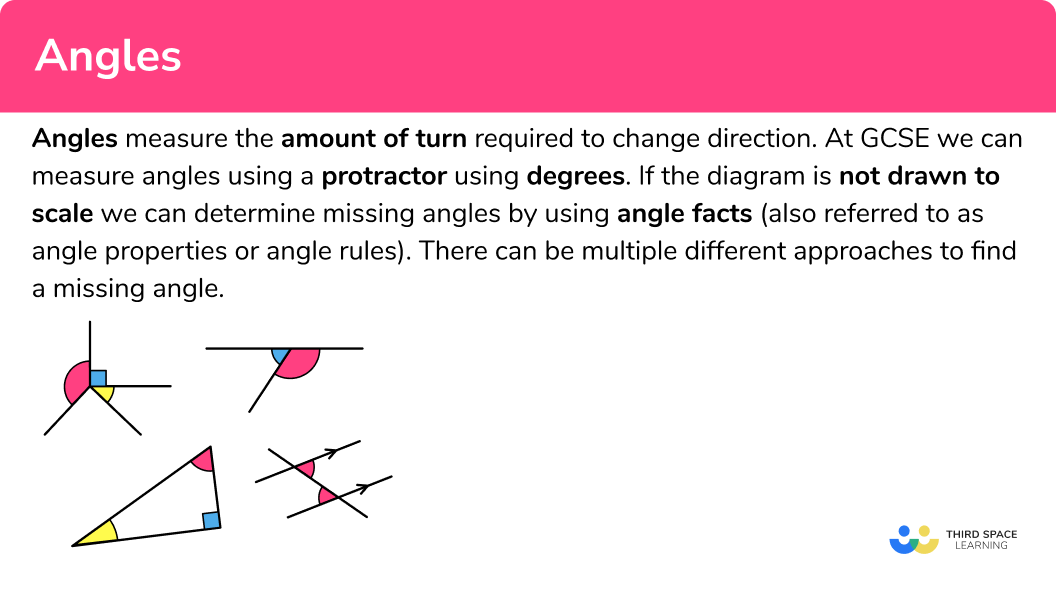### Angle types

There are different types of angles.

Step-by-step guide: Types of angles

### Angle rules

We can use angle rules to work out missing angles.

Angle rules are facts that we can apply to calculate missing angles in a diagram.

The five key angle facts that are used widely within the topic are:

• Angles on a straight line

The sum of angles on a straight line is always equal to \bf{180^{o}.}

A straight line would be considered to be half of a full turn; if you were standing on the line facing towards one end, you would have to turn 180 degrees to face the other end of the line.

A straight line can be called a straight angle if there is a vertex on the line and the turn around that vertex is 180^{o}.

• Angles at a point

The sum of angles at a point is always equal to \bf{360^{o}} .

A point would be considered to be a full turn; if you were standing at the point facing in one direction, you would have to turn 360 degrees to return back to your original position.

• Complementary angles

The sum of complementary angles is always equal to \bf{90^{o}} .

Complementary angles therefore make up a right angle.

These angles do not need to be together and form a right angle. If any two angles sum to 90^o they are complementary.

• Supplementary angles

The sum of supplementary angles is always equal to \bf{180^{o}} .

Supplementary angles therefore make up a straight line.

These angles do not need to be together on a straight line. If any two angles sum to 180^o they are supplementary.

• Vertically opposite angles

Vertically opposite angles are equal.

This occurs when two straight lines meet (intersect) at a point known as a vertex, forming an x shape where the opposite pairs of angles are the same size. Also, two adjacent angles are supplementary (they add to equal 180^o ).

Step-by-step guide: Angle rules

### Angles in polygons

We can calculate the interior and exterior angles of any polygon.

• Interior angles

The sum of interior angles in any n -sided shape is determined using the formula,

\text{Sum of interior angles}=180(n-2).

One interior angle of a regular polygon with n -sides is determined using the formula,

\theta=\frac{180(n-2)}{n}.

For an irregular polygon, the missing angle is calculated by subtracting all of the known angles from the total sum of the interior angles of the polygon.

• Exterior angles

The sum of exterior angles for any polygon is \bf{360^{o}} .

Whereas the interior angle sum is different for each n -sided shape, the exterior angle sum is always 360^{o}, regardless of how many sides the polygon has.

This is because, as you walk around the perimeter of the shape, the exterior angle is the turn from the direction of one edge to the next edge of the polygon.

For a regular polygon, each exterior angle is equal to 360 divided by the number of sides, n, and so

\text{Exterior angle}=\frac{360}{n}.

For an irregular polygon, the unknown exterior angle is calculated by subtracting the known exterior angles from 360^{o}.

The sum of an exterior angle and its adjacent interior angle is 180^{circ}, because they both lie on a straight line.

• Angles in a triangle

The sum of angles in a triangle is \bf{180^{o}} .

x + y + z = 180^o

Remember that there are four different types of triangles, each with a specific angle property.

The sum of angles in a quadrilateral is \bf{360^{o}} .

Any quadrilateral can be constructed from two adjacent triangles. This means that as the angle sum of a triangle is equal to 180^{o}, two triangles would have an angle sum of 360^{o}.

w +x + y + z= 180^o

There are several different types of quadrilaterals, each with a specific angle property.

Step-by-step guide: Angle in polygons

### Angles in parallel lines

Angles in parallel lines are facts that can be applied to calculate missing angles within a pair of parallel lines. The three key angle facts that are used when looking at angles in parallel lines are,

• Corresponding angles
• Alternate angles
• Co-interior angles

• Corresponding angles

Corresponding angles are equal.

When we intersect a pair of parallel lines with a transversal (another straight line), corresponding angles are the angles that occur on the same side of the transversal line. They are either both obtuse or both acute.

• Alternate angles

Alternate angles are equal.

When we intersect a pair of parallel lines with a transversal, alternate angles occur on opposite sides of the transversal line. They are either both obtuse or both acute.

• Co-interior angles

The sum of two co-interior angles is \bf{180^{o}} .

Co-interior angles occur in between two parallel lines when they are intersected by a transversal. The two angles that occur on the same side of the transversal always add up to 180^{o}.

Step-by-step guide: Angles in parallel lines

## How to use angles

We can use angles in lots of different contexts.

• Types of angles
• Angle rules
• Angles in polygons
• Angles in parallel lines

### Explain how to use angles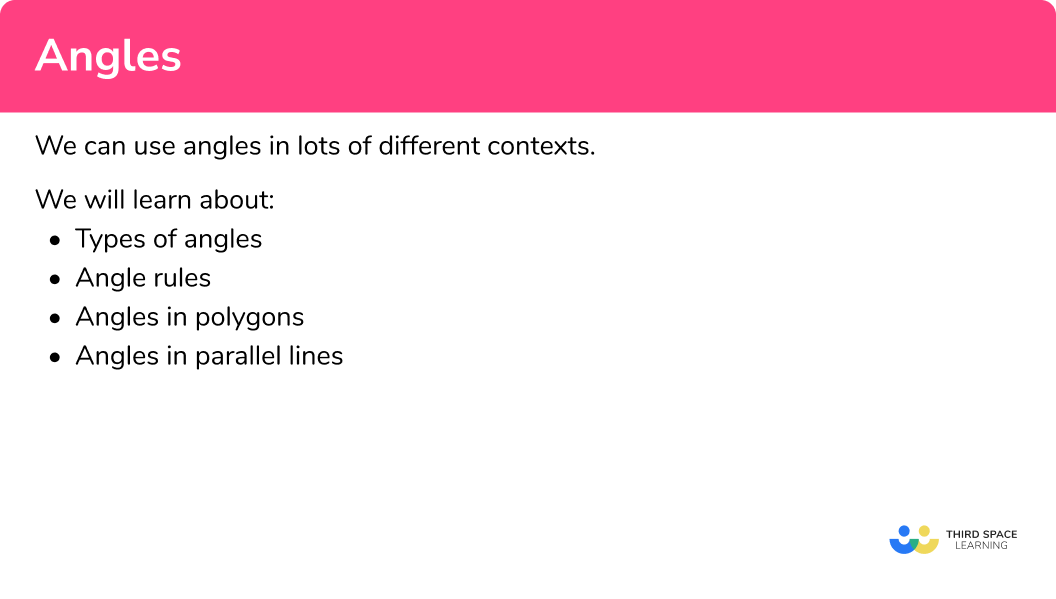## Angle rules examples

### Example 1: angles on a straight line

Calculate the value of x.

The angle highlighted as a square is a right angle. A right angle measures 90^{o}. Adding the angles together, we can form the expression

70+90=160.

2Subtract the angle sum from \bf{180^{o}} .

As the sum of angles on a straight line total 180^{o},

180-160=20.

3Form and solve the equation.

Here we have no equation to solve as the missing angle is 20^{o}.

### Example 2: angles at a point

Calculate the value of x.

Subtract the angle sum from \bf{360^{o}} .

Form and solve the equation.

### Example 3: complementary angles

Angle AOB is complementary to BOC. Determine the size of the angle AOB.

Identify which angles are complementary.

Clearly identify which of the unknown angles the question is asking you to find the value of.

Solve the problem and give reasons where applicable.

Clearly state the answer using angle terminology.

### Example 4: supplementary angles

Given that AC is a straight line, determine the size of angle AOB.

Identify which angles are supplementary.

Clearly identify which of the unknown angles the question is asking you to find the value of.

Solve the problem and give reasons where applicable.

Clearly state the answer using angle terminology.

### Example 5: vertically opposite angles

Given that AC and BD are straight intersecting lines at the point O, determine the size of angle COD.

Identify which angles are vertically opposite to one another.

Clearly identify which of the unknown angles the question is asking you to find the value of.

Solve the problem and give reasons where applicable.

Clearly state the answer using angle terminology.

### Example 6: interior angles of a regular pentagon

What is the interior angle sum of a regular pentagon?

Identify how many sides the polygon has.

Identify if the polygon is regular or irregular.

If possible work out how many triangles could be created within the polygon by drawing lines from one vertex to all other vertices.

Multiply the number of triangles by \bf{180} to calculate the sum of the interior angles.

### Example 7: angles in a triangle

What type of triangle is ABC?

Add up the other angles within the triangle.

Subtract this total from \bf{180^{o}} .

### Example 8: angles in a quadrilateral

ABCD is a kite. Calculate the size of angle ADO.

Use angle properties to determine any interior angles.

Subtract the angle sum from \bf{360^{o}} .

Form and solve the equation.

### Example 9: exterior angles

Calculate the exterior angle x for the hexagon below.

Identify the number of sides in any polygon given in the question.

Identify what the question is asking.

Solve the problem using the information you have already gathered.

### Example 10: alternate angles

Calculate the size of the angle x.

Highlight the angle(s) that you already know.

State the alternate angle, co-interior angle or corresponding angle fact to find a missing angle in the diagram.

Use basic angle facts to calculate the missing angle.

### Example 11: corresponding angles

Calculate the value of y.

Highlight the angle(s) that you already know.

State the alternate angle, co-interior angle or corresponding angle fact to find a missing angle in the diagram.

Use basic angle facts to calculate the missing angle.

State the alternate angle, co-interior angle or corresponding angle fact to find a missing angle in the diagram.

### Example 12: co-interior angles

Calculate the value of x.

Highlight the angle(s) that you already know.

State the alternate angle, co-interior angle or corresponding angle fact to find a missing angle in the diagram.

Repeat this process until the required missing angle is calculated.

### Common misconceptions

• Measuring angles with a protractor when the diagrams are not drawn accurately

When the question states that the diagram is not drawn accurately we cannot simply measure the missing angles using a protractor. We need to use angle facts to calculate the missing angle.

• Angles on a straight line and vertically opposite angle rules

With the straight line rule that only adjacent angles are considered, and for vertically opposite angles the lines must be straight.

• Exterior angles of a polygon

Exterior angles of a polygon have to travel in the same direction for the sum to be 360^{o}.

• Angles in triangles

Pairing up the incorrect angles in an isosceles triangle and using exterior angles when calculating the interior angle sum of a triangle.

### Practice angles questions

1. Calculate the value of x.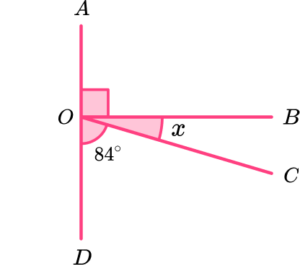6^o84^o90^o186^oThe sum of angles on a straight line is 180^{o}.

x=180-(90+84)=6^{o}

2. Calculate the value of x.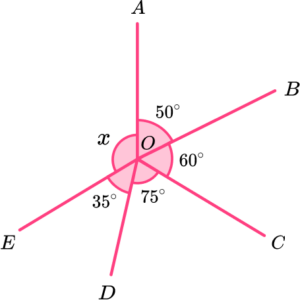120^o130^o140^o145^oThe sum of angles at a point is 360^{o}.

x=360-(50+60+75+35)=140^{o}

3. AOC is a right angle. Calculate the value of angle BOC.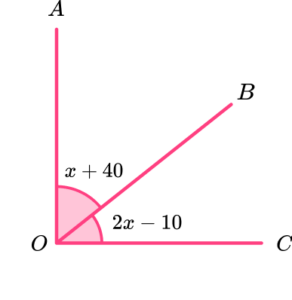80^o30^o60^o2x-10^oThe sum of two complementary angles is 90^{o}.

\begin{aligned} x+40+2x-10&=90\\ 3x+30&=90\\ 3x&=60\\ x&=20 \end{aligned}

2x-10=2\times{20}-10=30^{o}.

4. AB is a straight line. Calculate the size of angle AOC.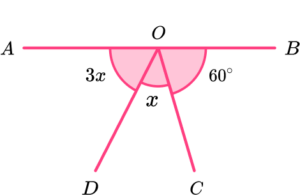30^o60^o90^o120^oAs AB is a straight line, angle AOC is supplementary to angle BOC.

Angle AOC = 3x+x=4x and so

4x=180-60=120

x=30^{o}.

5. The two straight lines AC and BD intersect at the point O. Calculate the value of x.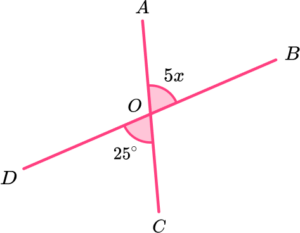5253120As AC and BD are straight lines, angle COD is vertically opposite angle AOB.

As vertically opposite angles are equal, we can form the equation

\begin{aligned} 5x&=25\\\\ x&=25\div{5}\\\\ x&=5. \end{aligned}

6. The hexagon below has two lines of symmetry. Calculate the size of the interior angle at A.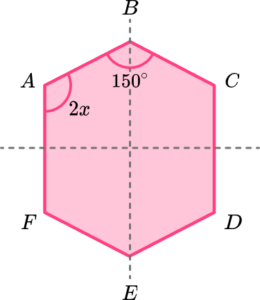15^o30^o105^o150^oS_{6}=180(6-2)=180\times{4}=720.

The interior angle at C = 2x given the vertical line of symmetry.

The interior angle at D,E, and F can be found using the horizontal line of symmetry: D = 2x, \ E = 150^{o}, and F = 2x.

The sum of angles in the hexagon is therefore

\begin{aligned} 2x+150+2x+2x+150+2x&=720\\\\ 8x+300&=720\\\\ 8x&=420\\\\ x&=52.5 \end{aligned}

As the interior angle at A is equal to 2x,

A =2x=2\times{x}=2\times{52.5}=105^{o}.

7. Calculate the value of y.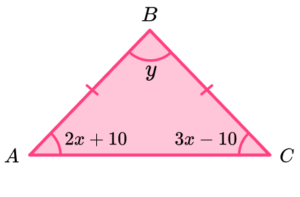60^o45^o90^o80^o\begin{aligned} 3x-10&=2x+10\\\\ 3x&=2x+20\\\\ x&=20 \end{aligned}

2x+10=2\times{20}+10=50

3x-10=3\times{20}-10=50

As the sum of angles in a triangle is 180^{o},

y=180-(50+50)=80^{o}.

8. Calculate the interior angle at A.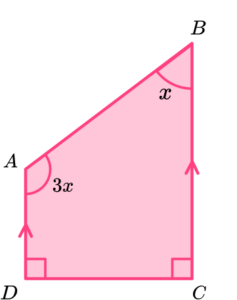30^o45^o90^o135^oThe sum of angles in a quadrilateral is 360^{o}.

\begin{aligned} 3x+x+90+90&=360\\\\ 4x+180&=360\\\\ 4x&=180\\\\ x&=45 \end{aligned}

3x=3\times{45}=105^{o}

9. Calculate the interior angle of a regular octagon.

22.5^o45^o135^o180^oS_{8}=180(8-2)=180\times{6}=1080.

1080\div{8}=135^{o}

10. Which diagram only needs us to use alternate angles to determine the value of x?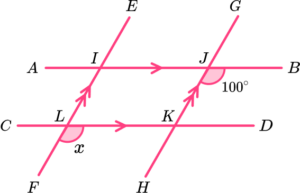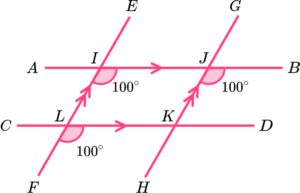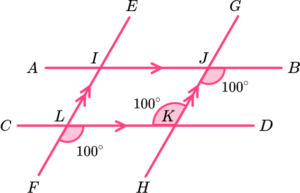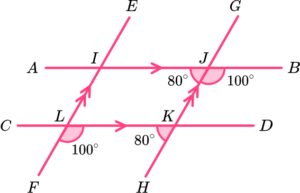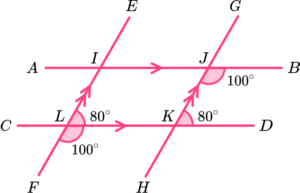BJH is alternate to JKL.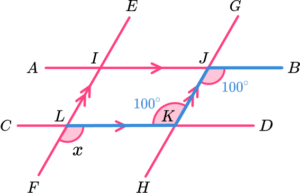JKL is alternate to KLF.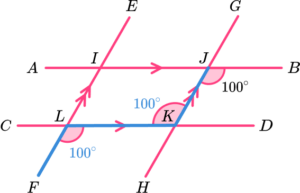11. Which diagram needs us to use corresponding angles to determine the value of x?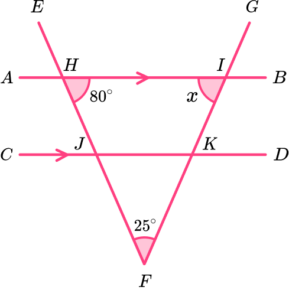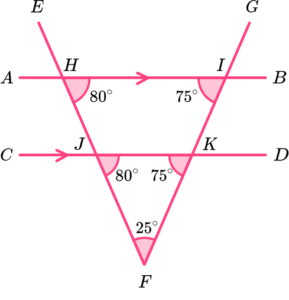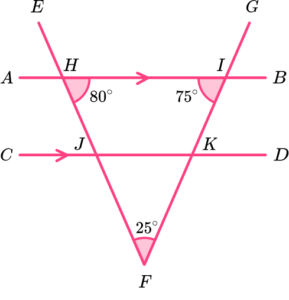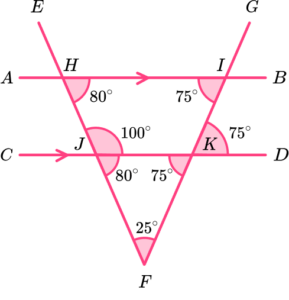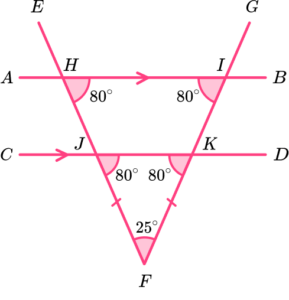BJH is corresponding to KJF.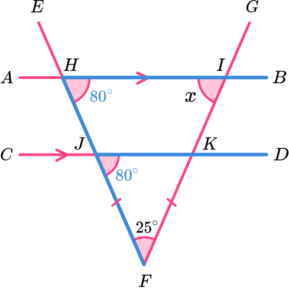The sum of angles in a triangle is 180^o and so angle

JKF = 180-(80 + 25) = 75.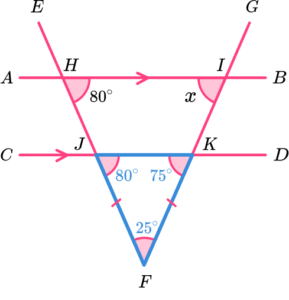Angle JKL is corresponding to HIK.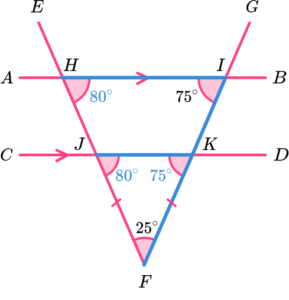12. Which diagram uses co-interior angles to calculate the value of x?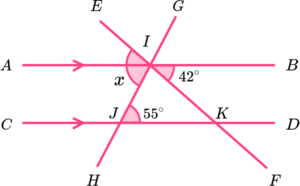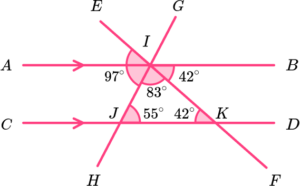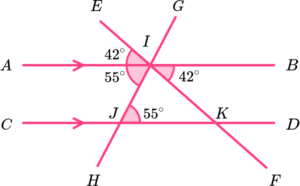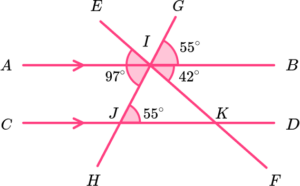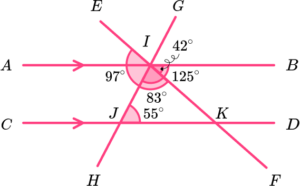DJI is co-interior to BIJ.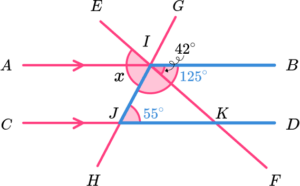125-42 = 83.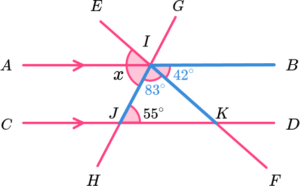EF is a straight line. The sum of angles on a straight line is 180^{o}.

180-83 = 97^{o}.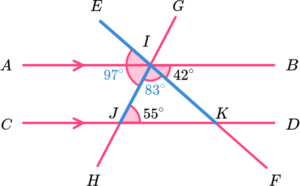### Angles GCSE questions

1. A sock design requires four colours of thread. The pie chart below shows the proportion of each colour.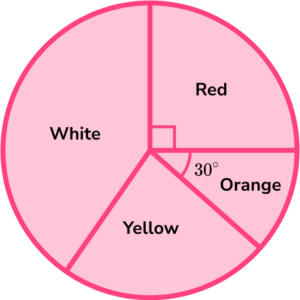The ratio of white to yellow is 2:1. What fraction of the thread is white?

(4 marks)

360-(90+30)=240

(1)

240 \div (2+1)=80

(1)

80 \times 2=160^o

(1)

\frac{160}{360}

(1)

2. ABC and ACD are two congruent isosceles triangles.

Show that the angle BCD is double the angle BAD.

State any angle rules you use.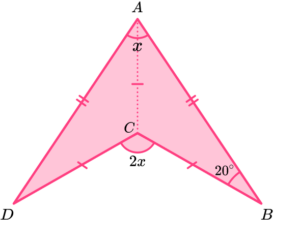(5 marks)

BAC = ABC = 20^o and base angles in an isosceles triangle are equal.

(1)

ACB = 180-(20 + 20) = 140^o and the sum of angles in a triangle is 180^{o}.

(1)

ACD = ACB = 140^o and the two triangles are congruent.

(1)

BAD = 20 + 20 = 40^o and the two triangles are congruent.

(1)

BCD = 360-(140 + 140) = 80^o and the sum of angles at a point is 360^{o}.

(1)

3. Below are the three lines AB, \ CD, and EF, intersected by the line GH.

Show that the lines AB, \ CD, and EF are not parallel.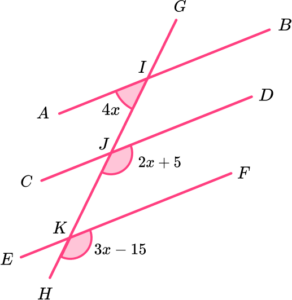(7 marks)

Method 1

If AB and CD are parallel, 4x+2x+5=180.

(1)

DJK is corresponding to BIJ.

(1)

The sum of angles on a straight line total 180^{o}.

(1)

x=29.1\dot{6}

(1)

and

2x+5=3x-15

(1)

x=20

(1)

and

x must be the same for both equations.

(1)

Alternative Method

If AB and EF are parallel, 4x+3x-15=180.

(1)

FKH is corresponding to BIJ.

(1)

The sum of angles on a straight line total 180^{o}.

(1)

x=27.86 \ (2dp)

(1)

and

2x+5=3x-15

(1)

x=20

(1)

and

x must be the same for both equations.

(1)

## Learning checklist

You have now learned how to:

• Recognise angles as a property of shape or a description of a turn
• Apply the properties of angles at a point, angles at a point on a straight line, and vertically opposite angles
• Derive and use the sum of angles in a triangle and use it to deduce the angle sum in any polygon, and to derive properties of regular polygons
• Distinguish between regular and irregular polygons based on reasoning about equal sides and angles
• Understand and use the relationship between parallel lines and alternate and corresponding angles

## Still stuck?

Prepare your KS4 students for maths GCSEs success with Third Space Learning. Weekly online one to one GCSE maths revision lessons delivered by expert maths tutors.

Find out more about our GCSE maths tuition programme.